# OECD Business confidence Index(BCI) data analysis 8 - BCI and GDP Growth are positively correlated.Photo by J Lee on Unsplash

This post is following of above post.
In the previous post, I made panel data dataframe.
Let's analyze this.

Firstly, let's see correlation.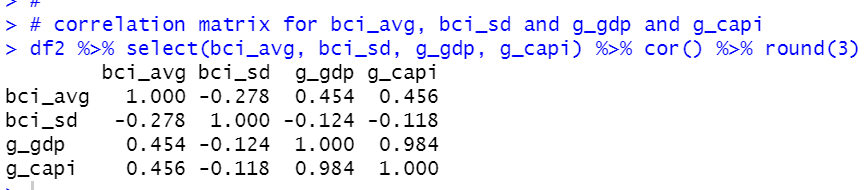g_gdp and g_capi are highly correlated. bci_sd are negatively correlated to others.

Let's make a linear regression model that explaines bci_avg with bci_sd and g_gdp.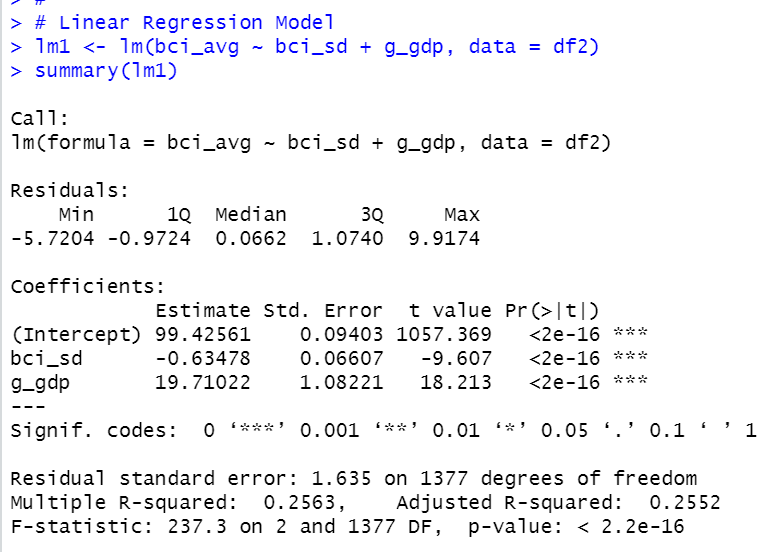If bci_sd increase by 1, bci_avg decrease by -0.63. If g_gdp increase by 0.01, bci_avg increase by 0.197.

Let's check if there is heteroskedasticity for error terms.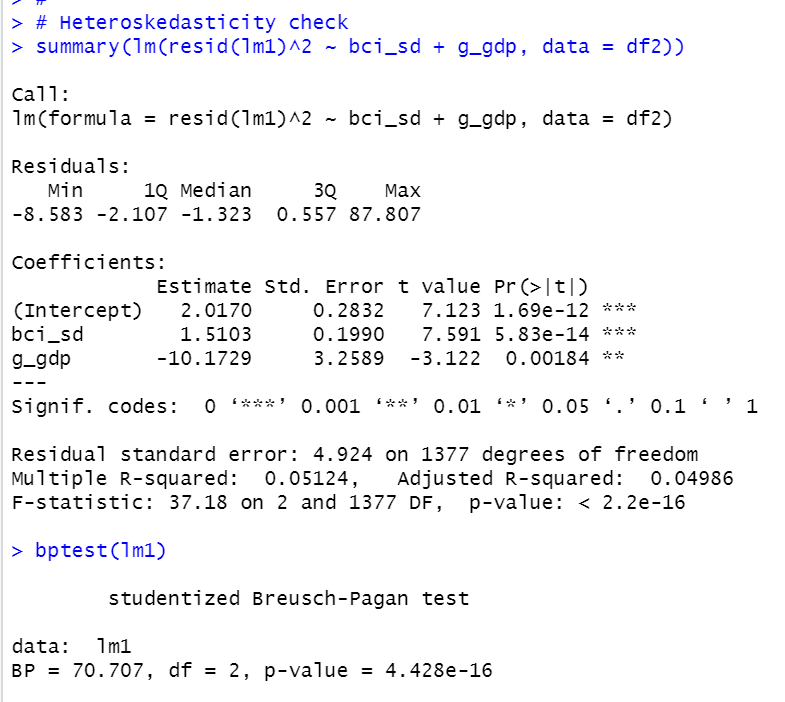I did manuall checking and using bptest(). The both meshod shows there is heteroskedasticity.

Let's see residuals plot chart.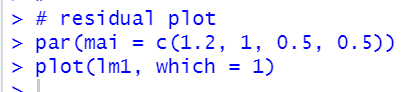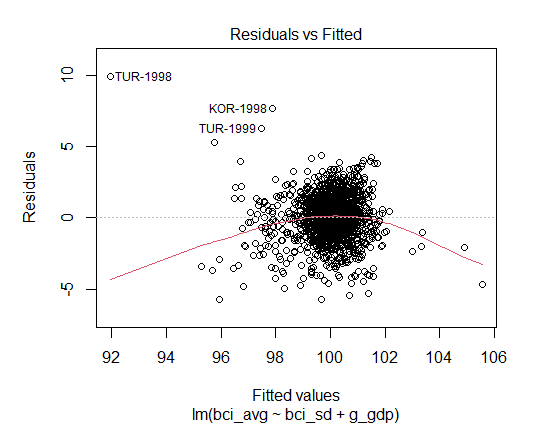Since there is heteroskedasticity, we need heteroskedasticity-robust SE.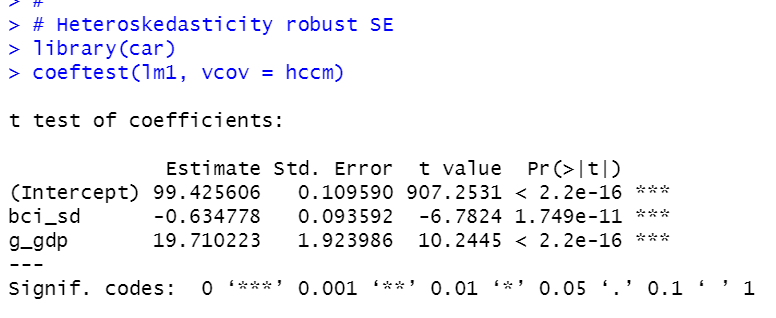Let's see how well lm1 model matches actual data for JPN data.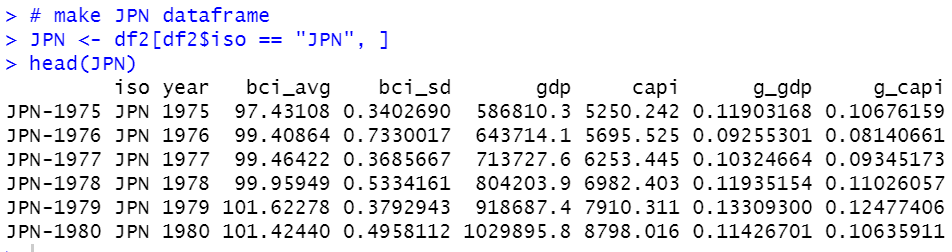2. I made predicted bci_avg with predict() function.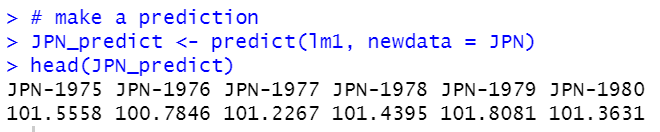3. plot actual bci_avg and predicted value.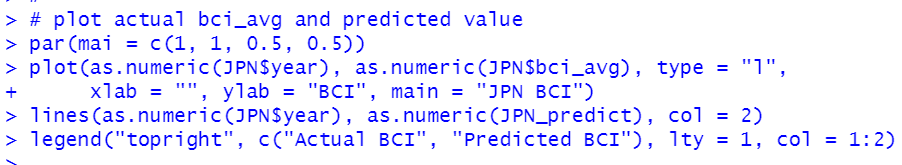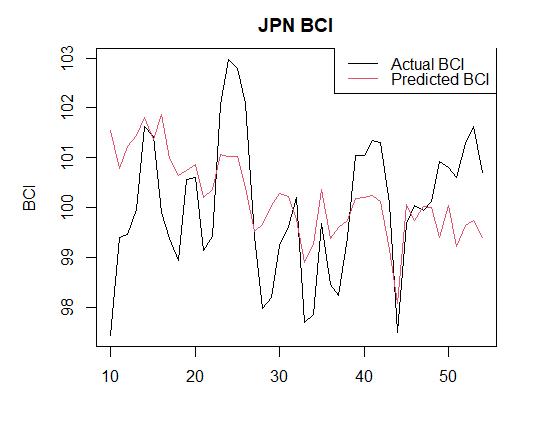It seems not good fitting.

That's it．Thank you!

To read from the 1st post,